###### FREE Expert Solution

This problem involves rotational kinematics. The approach is simple and straightforward.

Angular acceleration is expressed as:

$\overline{){\mathbf{\alpha }}{\mathbf{=}}\frac{\mathbf{\tau }}{\mathbf{I}}}$, where τ is torque and I is the moment of inertia.

Part A)

Work done:

$\overline{){W}{=}{\tau }{∆}{\theta }}$

We're given τ = 25.0 N•m

I = 50.0 kg•m2

Therefore, the angular acceleration is:

80% (464 ratings)###### Problem Details

Consider a motor that exerts a constant torque of 25.0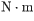to a horizontal platform whose moment of inertia is 50.0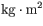. Assume that the platform is initially at rest and the torque is applied for 12.0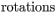. Neglect friction.

Part A ) How much work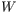does the motor do on the platform during this process?=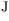Part B ) What is the rotational kinetic energy of the platform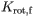at the end of the process described above?=Part C ) What is the angular velocity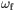of the platform at the end of this process?=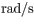Part D ) How long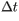does it take for the motor to do the work done on the platform calculated in Part A?=Part E ) What is the average power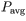delivered by the motor in the situation above?=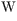Part F ) Note that the instantaneous power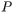delivered by the motor is directly proportional to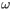, soincreases as the platform spins faster and faster. How does the instantaneous power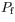being delivered by the motor at the time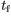compare to the average powercalculated in Part E?

Note that the instantaneous power delivered by the motor is directly proportional to , so increases as the platform spins faster and faster. How does the instantaneous power being delivered by the motor at the time compare to the average power calculated in Part E?

 a.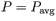b.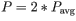c.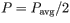d. none of the above